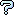All about flooble | fun stuff | Get a free chatterbox | Free JavaScript | Avatarsperplexus dot infoA Self Intersecting Curve (Posted on 2005-12-16)The curve defined by the relation x^3+y^3=3xy intersects itself at the origin and forms a loop. Find the area enclosed by the loop.

 Submitted by Brian Smith Rating: 4.5000 (2 votes) Solution: (Hide) The area enclosed by the loop is 3/2. Use the line x=y as the axis of integration. The intersection of the curve and x=y are (0,0) and (3/2,3/2). The length of the axis is 3/sqrt(2). Define d to be the distance along x=y starting from (0,0). At d=3/sqrt(2) the point (3/2, 3/2) is reached. The set of lines perpendicular to x=y form the cross-sections of integration. At distance d, the cross-section is the segment on the line x+y=d*sqrt(2). The endpoints of the segment can be found by solving the system {x^3+y^3=3xy, x+y=d*sqrt(2)} x^3+y^3=3xy can be rewritten as (x+y)^3=3xy(x+y+1) After substituting y=d*sqrt(2)-x: 2d^3*sqrt(2) = 3x(d*sqrt(2)-x)(1+d*sqrt(2)) Expressing as a quadratic in x: (3+3d*sqrt(2))x^2 + (-3d*sqrt(2)-6d^2)x + (2d^3*sqrt(2)) = 0 Solving for x gives: x = ( (3d*sqrt(2)+6d^2) +\\- sqrt( (3d*sqrt(2)+6d^2)^-4*(2d^3*sqrt(2))(3+3d*sqrt(2)) ) )/( 6+6d*sqrt(2) ) Simplifying: x = ( (3d*sqrt(2)+6d^2) +\\- sqrt( 18d^2+12d^3*sqrt(2)-12d^4 ) )/( 6+6d*sqrt(2) ) Denoting the two roots as x1 and x2, the endpoints are (x1,x2) and (x2,x1) The length of the segment is then |x2-x1|*sqrt(2), which equals: 2d*sqrt(9+6d*sqrt(2)-6d^2)/(3+3d*sqrt(2)) The integral which will calculate the area of the loop is then: Integ{0,3/sqrt(2)}[2d*sqrt(9+6d*sqrt(2)-6d^2)/(3+3d*sqrt(2))]dd First make the substitution u=d*sqrt(2) to simplify the expression: u=d*sqrt(2), du=sqrt(2)dd, d=0 -> u=0, d=3/sqrt(2) -> u=3 Integ{0,3}[u*sqrt(9+6u-3u^2)/(3u+3)]du Before the next substitution, rewrite the integral as: Integ{0,3}[u*sqrt(4-(u-1)^2)/((u+1)*sqrt(3))]du Now, make the substitution u-1=2*sin(T) u-1=2*sin(T), du=2*cos(T)dT, u=0 -> T=-pi/6, u=3 -> T=pi/2 Integ{-pi/6,pi/2}[(2*sin(T)+1)*sqrt( 4-4*(sin(T))^2 )/( (2+2*sin(T) )*sqrt(3))]dT The integral simplifies to: (2/sqrt(3))*Integ{-pi/6,pi/2}[sin(T)+cos(2T)]dT The integrand evaluates to (1/2)sin(2T)-cos(T) and the whole integral evaluates to (2/sqrt(3))*(0 + 0 + sqrt(3)/2 + (1/2)*(sqrt(3)/2)) = 3/2.Comments: ( You must be logged in to post comments.)
 Subject Author DateAnswer K Sengupta 2008-03-19 11:06:39re: Numerical Integration Solution Richard 2005-12-17 13:31:23Another numerical method as solution Charlie 2005-12-17 11:33:49Numerical Integration Solution Richard 2005-12-17 02:44:28re(3): A solution; Not quite Richard 2005-12-16 14:09:55re(3): A solution; oops Larry 2005-12-16 12:20:10re(2): A solution; Not quite goFish 2005-12-16 11:59:29re: A solution; Not quite Larry 2005-12-16 11:41:27A solution goFish 2005-12-16 11:12:50Please log in:
 Login: Password: Remember me: Sign up! | Forgot password

 Search: Search body:
Forums (0)
Newest Problems
Random Problem
FAQ | About This Site
Site Statistics
New Comments (1)
Unsolved Problems
Top Rated Problems
This month's top
Most Commented On

Chatterbox:
Copyright © 2002 - 2019 by Animus Pactum Consulting. All rights reserved. Privacy Information